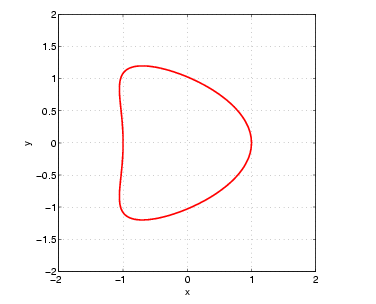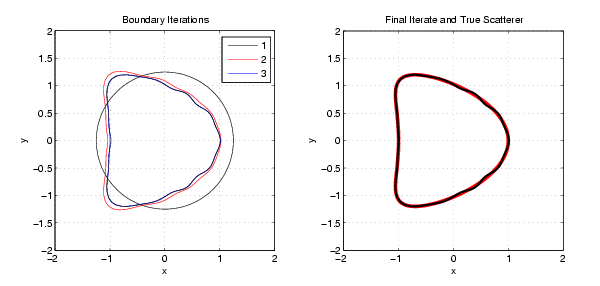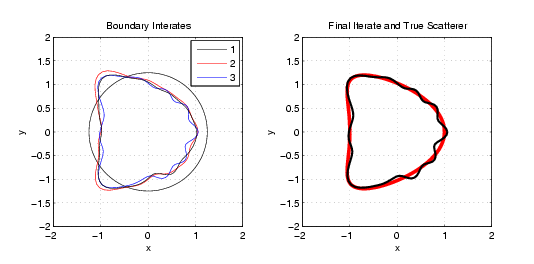# Inverse Acoustic Scattering in 2D

The publication that is linked to this page is a relatively extensive study of two dimensional inverse acoustic scattering algorithms for problems with full aperture data (the object is viewed from all angles) and partial aperture data (the object is viewed only over a restricted set of aspect angles). The implemented algorithms include:
• Full aperture quasi-newton Fourier based inverse backscattering
• Partial aperture quasi-newton collocation based inverse backscattering
• Full or partial aperture secant based inverse backscattering
• Full or partial aperture full derivative based inverse backscattering
These were implemented for both forward scattering: the incident wave is from one direction and the receive aperture is somewhere else, and backscattering: the incident and receive aperture are located at the same point in space. Below you will find some pictures showing the initial scatter and its reconstructions using various algorithmic techniques. Please email with any questions/comments you might have.The "kite" scatter we will use as our base object to scatter waves from and reconstruct.The monostatic boundary iterates and the true kite-shaped scatterer for:
k=1, NF = 11, R_0 = 1.2, N_theta = 201, N_pD = 100, and Gamma = [ 0 , 2\pi].The monostatic boundary iterates and the true kite-shaped scatterer for:
k=1, NF = 11, R_0 = 1.2, N_theta = 201, N_pD = 100, and Gamma = [ 0, \pi/2]
John Weatherwax